# Hexrays Toolbox

Hexrays Toolbox (hxtb) is a powerful script for the Hexrays Decompiler which can be used to find code patterns in decompiled code. Some of its use cases are described below.

## Use Cases

• scan binary files for known and unknown vulnerabilities
• locate code patterns from previously reverse engineered executables in newly decompiled code
• malware variant analysis
• find code similarities across several binaries
• find code patterns from one architecture in executable code of another architecture
• many more, limited (almost) only by the queries you'll come up with ;)

The query shown below shows an example of how a particular code pattern can be identified that was responsible for a remote code execution security vulnerability in WHatsApp in 2019 (CVE-2019-3568, libwhatsapp.so). Find the example script.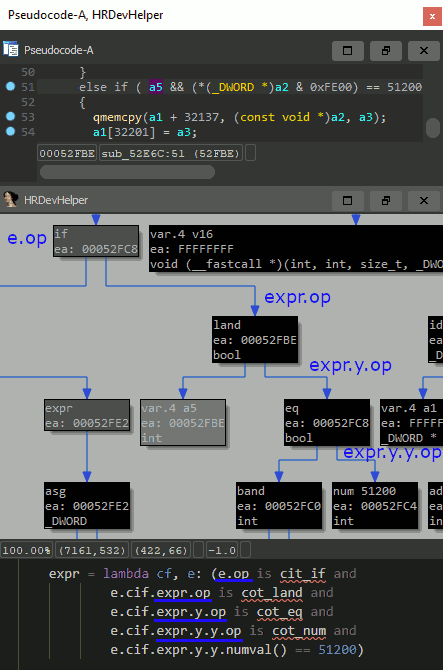## Usage

There are several ways of using Hexrays Toolbox, each with a varying degree of flexibility.

• run queries on behalft of hxtb_shell, an interactive GUI
• custom scripting
• `interactive.py`, a script that adds convenience functions to be used with the IDA command line interface
• `automation.py`, a script that processes and runs hxtb queries on a given set of files in batch mode

### hxtb-shell

Executing the included `hxtb_shell.py` script from within IDA opens a GUI window that can be used to develop and run hxtb queries with. hxtb-shell also accepts Python expressions created by the HRDevHelper plugin's context viewer that can be directly copy-pasted.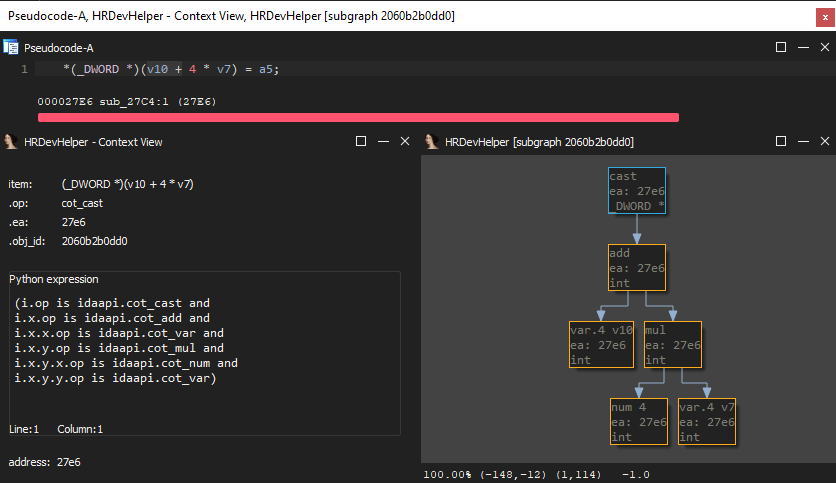Below screenshot shows hxtb-shell with an example query loaded that creates and shows a list of strings referenced by the current function.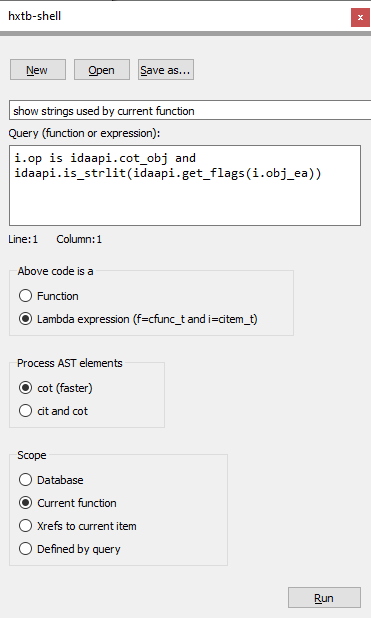Further queries in the hxtb-shell format can be found in the `hxtbshell_queries` folder included with hxtb.

### Scripting

Loading `hxtb.py` with IDA (alt-f7) makes functions such as `find_expr()` and `find_item()` available to both the IDAPython CLI and the script interpreter (shift-f2). Among others, these functions can be used to run queries on the currently loaded ida database. Please check out some of the examples shown below.

``````    find_item(ea, q)
find_expr(ea, q)

Positional arguments:
ea:         address of a valid function within
the current database
q:          lambda function
custom lambda function with the following arguments:
1. cfunc: cfunc_t
2. i/e:   cinsn_t/cexpr_t
Returns:
list of query_result_t objects

Example:
find_expr(here(), lambda cf, e: e.op is cot_call)

-> finds and returns all function calls within a current function.
The returned data is a list of query_result_t objects (see hxtb.py).

The returned list can be passed to an instance of the ic_t class,
which causes the data to be displayed by a chooser as follows:

from idaapi import *
import hxtb
hxtb.ic_t(find_expr(here(), lambda cf,e:e.op is cot_call))

Please find the cfunc_t, citem_t, cinsn_t and cexpr_t structures
within hexrays.hpp for further help and details.
``````

Please also check out the HRDevHelper plugin and the IDAPyHelper script which may assist in writing respective queries.

## Examples

### get list of expressions that compare anything to zero ("x == 0")

``````         cot_eq
/   \
x /     \ y
(anything)  cot_num --- n.numval() == 0
``````
``````from idaapi import *
from hxtb import find_expr
query = lambda cfunc, e: e.op is cot_eq and e.y.op is cot_num and e.y.numval() == 0
r = find_expr(here(), query)
for e in r:
print(e)
``````

### get list of function calls

``````        cot_call
/
x /
cot_obj
``````
``````from idaapi import *
from hxtb import find_expr
query = lambda cfunc, e: e.op is cot_call and e.x.op is cot_obj
r = find_expr(here(), query)
for e in r:
print(e)
``````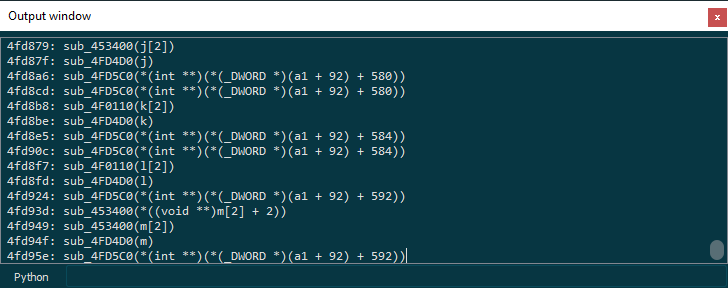### print list of memcpy calls where "dst" argument is on stack

``````        cot_call --- arg1 is cot_var
/           arg1 is on stack
x /
cot_obj --- name(obj_ea) == 'memcpy'
``````
``````from idaapi import *
from hxtb import find_expr
r = []
query = lambda cfunc, e: (e.op is cot_call and
e.x.op is cot_obj and
get_name(e.x.obj_ea) == 'memcpy' and
len(e.a) == 3 and
e.a.op is cot_var and
cfunc.lvars[e.a.v.idx].is_stk_var())
for ea in Functions():
r += find_expr(ea, query)
for e in r:
print(e)
``````

### get list of calls to sprintf(str, fmt, ...) where fmt contains "%s"

``````        cot_call --- arg2 ('fmt') contains '%s'
/
x /
cot_obj --- name(obj_ea) == 'sprintf'
``````
``````from idaapi import *
from hxtb import find_expr
r = []
query = lambda cfunc, e: (e.op is cot_call and
e.x.op is cot_obj and
get_name(e.x.obj_ea) == 'sprintf' and
len(e.a) >= 2 and
e.a.op is cot_obj and
b'%s' in get_strlit_contents(e.a.obj_ea, -1, 0, STRCONV_ESCAPE))
for ea in Functions():
r += find_expr(ea, query)
for e in r:
print(e)
``````

### get list of signed operators, display result in chooser

``````from idaapi import *
from hxtb import ic_t
query = lambda cfunc, e: (e.op in
[hr.cot_asgsshr, hr.cot_asgsdiv,
hr.cot_asgsmod, hr.cot_sge,
hr.cot_sle, hr.cot_sgt,
hr.cot_slt, hr.cot_sshr,
hr.cot_sdiv, hr.cot_smod])
ic_t(query)
``````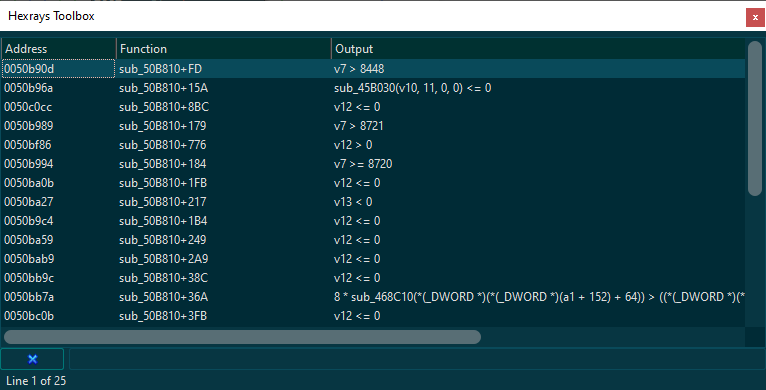### get list of "if" statements, display result in chooser

``````from idaapi import *
from hxtb import ic_t
ic_t(lambda cf, i: i.op is cit_if)
``````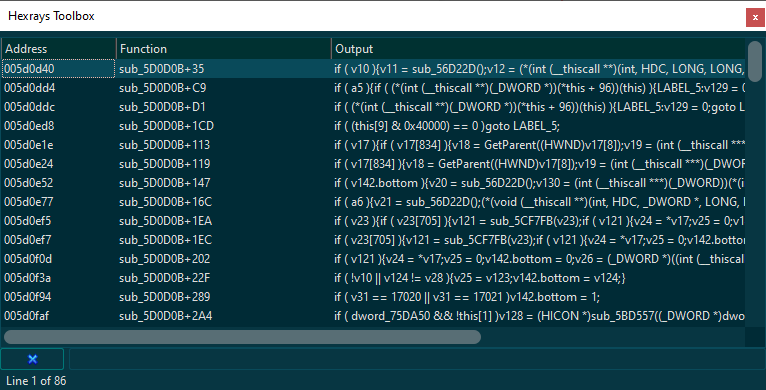### get list of all loop statements in db, display result in chooser

``````from idaapi import *
from hxtb import ic_t, query_db
ic_t(query_db(lambda cf,i: is_loop(i.op)))
``````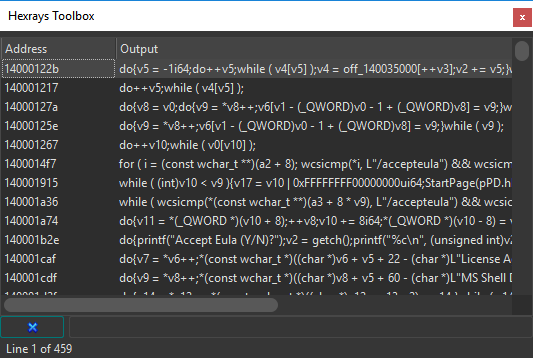### get list of loop constructs containing copy operations

``````from hxtb import ic_t, query_db, find_child_expr
from ida_hexrays import *

find_copy_query = lambda cfunc, i: (i.op is cot_asg and
i.x.op is cot_ptr and
i.y.op is cot_ptr)

find_loop_query = lambda cfunc, i: (is_loop(i.op) and
find_child_expr(cfunc, i, find_copy_query))

ic_t(query_db(find_loop_query))
``````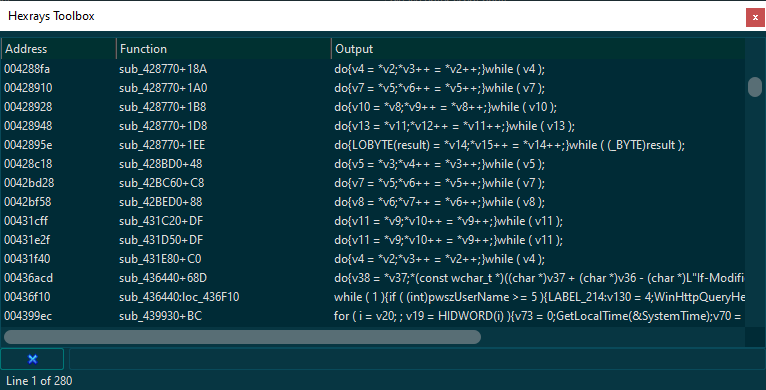Get A Weekly Email With Trending Projects For These Topics
No Spam. Unsubscribe easily at any time.
Python (1,116,833
Reverse Engineering (1,988
Ast (872
Loop (337
Pattern Matching (302
Decompiler (168
Ida Pro (158
Related Projects